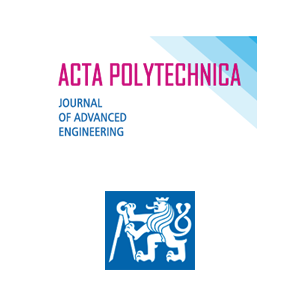Oksana Lytvyniak

# Deformation analysis of layered reinforced concrete - foam concrete floor slabs

Číslo: 4/2020
Periodikum: Acta Polytechnica
DOI: 10.14311/AP.2020.60.0324

Klíčová slova: Stress-strain state, deformation analysis, floor slab, foam concrete, normal concrete, reinforcement rod, distribution diagram of relative deformations, layered reinforced concrete - foam concrete floor slab

Pro získání musíte mít účet v Citace PRO.

Anotace: This article presents a theoretical study of a stress-strain state of layered reinforced concrete - foam concrete floor slabs (hereinafter called as the LRFCS), with the use of a deformation analysis. Compressive and tensile diagrams of the foam concrete, a tensile diagram of the reinforced concrete and compressive and tensile diagrams of the reinforcement rod are used for the estimation of the stress-strain state of the calculated cross-section of the LRFCS. It should be noted that this article presents the deformation method of loading by the scheme of pure bending for the LRFCS. This deformation method of loading is determined by six shapes of the stress-strain state. These shapes of the stress-strain state are represented by the corresponding distribution diagrams of the relative deformations and the distribution diagrams of internal stresses in the calculated cross-section of the floor slab. Also, this article presents the corresponding equilibrium equations of internal efforts and moments, which act in the calculated cross-section of the floor slab for all shapes of its stress-strain state. Consequently, the mentioned recommendations and mathematical dependencies allow to evaluate the stress-strain state of the LRFCS from its initial loading to its destruction.